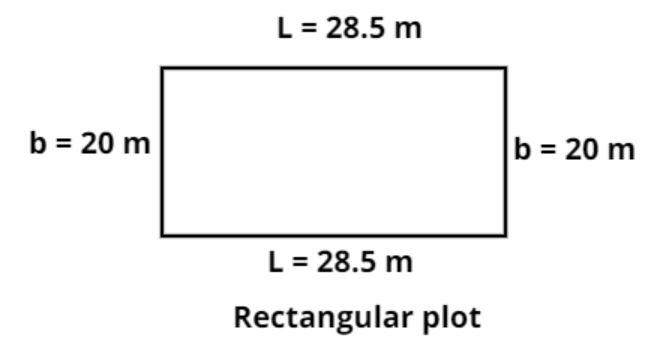QuestionAnswers

# Find the area and the perimeter of a rectangular plot of land whose length and breadth are 28.5 m and 20 m respectively.Hint – In this particular type of question use the concept that the area of the rectangular plot is the multiplication of the length and breadth of the rectangle and perimeter of any shape is the sum of all the side lengths of the shape so use these concepts to reach the solution of the question.Given data:
Length and breadth of the rectangular plot are 28.5 meter and 20 meter respectively.
Let the length be denoted by L and the breadth be denoted by B.
So, L = 28.5 meter
And, B = 20 meter.
The pictorial representation of the rectangular plot is shown in the above diagram.
Now as we know that in a rectangle the opposite sides are equal to each other.
So the length of the opposite side of the rectangle is also 28.5 meter and the breadth of the opposite side of the rectangle is also 20 meter as shown in the figure.
Now as we know that the perimeter of any shape is the sum of all the side lengths of the shape.
So the perimeter of the rectangle is the sum of all the sides of the rectangle.
Let perimeter be denoted by P.
Therefore, P = 2(L + B) = 2(28.5 + 20) = 2(48.5) = 97 meter.
So the perimeter of the rectangle is 97 meter.
Now as we know that the area (A) of the rectangle is the product of the length and the breadth of the rectangle.
So the area of the rectangle is
A = length $\times$ Breadth.
Now substitute the values we have,
Therefore, A = 28.5 $\times$ 20 = 570 square meter.
So this is the required answer.

Note – Whenever we face such types of questions the key concept we have to remember is that in the rectangle opposite sides are equal so mark the opposite sides of the rectangle then calculate the perimeter of the rectangle by adding all the sides and calculate the area of rectangle by multiplying length and breadth and simplify we will get the required answer.
View Notes
The Perimeter of Rectangle FormulaPerimeter of a Trapezoid FormulaArea of a Rhombus FormulaArea of a Sector of a Circle FormulaTo Find the Weight of a Given Body Using Parallelogram Law of VectorsArea of TrapeziumCBSE Class 8 Maths Chapter 11 - Mensuration FormulasSurface Area of a Prism FormulaArea of Hollow CylinderThe Making of a ScientistImportant Questions for CBSE Class 7 Maths Chapter 11 - Perimeter and AreaImportant Questions for CBSE Class 6 English A Pact with The Sun Chapter 8 - A Pact with the SunCBSE Class 8 Science Stars and The Solar System WorksheetsImportant Questions for CBSE Class 6 English A Pact with The Sun Chapter 6 - The Monkey and the CrocodileCBSE Class 8 Science Reaching The Age of Adolescence WorksheetsImportant Questions for CBSE Class 7 Social Science - Social and Political Life Chapter 8 - A Shirt In The MarketImportant Questions for CBSE Class 8 Social Science Our Past 3 Chapter 4 - Tribals, Dikus and the Vision of a Golden AgeImportant Questions for CBSE Class 8 Science Chapter 17 - Stars and The Solar SystemImportant Questions for CBSE Class 8 English Honeydew Chapter 1 - The Best Christmas Present in the WorldImportant Questions for CBSE Class 12 Chemistry Chapter 8 - The d and f Block ElementsCBSE Class 10 Hindi A Question Paper 2020Hindi A Class 10 CBSE Question Paper 2009Hindi A Class 10 CBSE Question Paper 2015Hindi A Class 10 CBSE Question Paper 2007Hindi A Class 10 CBSE Question Paper 2013Hindi A Class 10 CBSE Question Paper 2016Hindi A Class 10 CBSE Question Paper 2012Hindi A Class 10 CBSE Question Paper 2010Hindi A Class 10 CBSE Question Paper 2008Hindi A Class 10 CBSE Question Paper 2014NCERT Solutions for Class 7 Maths Chapter 11 Perimeter and AreaRS Aggarwal Class 6 Solutions Chapter-21 Concept of Perimeter and AreaNCERT Solutions for Class 7 Maths Chapter 11 Perimeter and Area In HindiRS Aggarwal Class 8 Mathematics Solutions for Chapter-18 Area of a Trapezium and a PolygonRS Aggarwal Class 10 Solutions - Perimeter and Areas of Plane FiguresNCERT Solutions for Class 7 Maths Chapter 11 Perimeter and Area (EX 11.4) Exercise 11.4NCERT Solutions for Class 7 Maths Chapter 11 Perimeter and Area (EX 11.2) Exercise 11.2RS Aggarwal Solutions Class 6 Chapter-21 Concept of Perimeter and Area (Ex 21C) Exercise 21.3NCERT Exemplar for Class 7 Maths Solutions Chapter 9 Perimeter & AreaNCERT Solutions for Class 7 Maths Chapter 11 Perimeter and Area (EX 11.3) Exercise 11.3